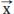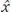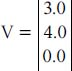NextPrevious

# What is a normalized vector?

A normalized (or unit) vector is one in which the sum of the squares of all coordinates is equal to one. For example, the vector (2, 2, 0) is not normalized; the vectors (0.707, 0.707, 0.0) and (1.0, 0.0, 0.0) are normalized. (An outward normal is another name for a normalized vector; it represents the direction that a polygon surface or vertex [end-point] is facing.) Normalized (or unit) vectors are often seen written as, but more usually as(theis often referred to as a “hat”).

A vector can be normalized by calculating the magnitude or length of the vector and dividing each coordinate by this value. For example, consider the following vector:Close

This is a web preview of the "The Handy Math Answer Book" app. Many features only work on your mobile device. If you like what you see, we hope you will consider buying. Get the App